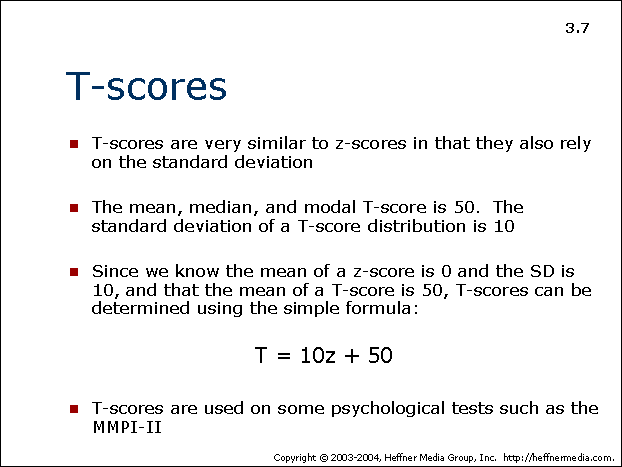# 07: T-Scores: Relying on Standard Deviation## T-scores

• T-scores are very similar to z-scores in that they also rely on the standard deviation
• The mean, median, and modal T-score is 50. The standard deviation of a T-score distribution is 10
• Since we know the mean of a z-score is 0 and the SD is 10, and that the mean of a T-score is 50, T-scores can be determined using the simple formula:

T = 10z + 50</p align = “center”>

• T-scored are used on some psychological tests such as MMPI-II